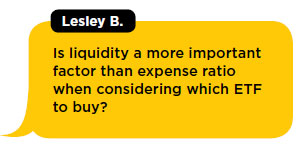# Digging Into Duration

October 22, 2019

ETF UNIVERSITY

As a general rule, bond prices go down when interest rates go up. But prices for some bonds fall more than others given the exact same change in interest rates. Understanding the basic drivers of interest rate risk can help you identify which bonds make the most sense for you, and can help you identify which bonds are best to own—or best to avoid—if you think interest rates will increase.

In general, bond values decrease when interest rates increase, because investors can get a better deal in a rising rate environment. Imagine a bond that’s fairly priced and paying a 4% coupon. That coupon—another word for the regular interest payment—is typically a fixed cash amount, say, 4% of a \$100 par bond, or \$4. If interest rates suddenly increase, a newly issued bond that’s very similar to the original bond might carry a 5% coupon, paying \$5 annually and automatically becoming more attractive than the bond paying 4%.

Understanding Duration
The statistic used to measure this time-weighted interest rate risk is called “duration.” A higher time-weighted average—a higher duration—means more interest rate risk, and clearly it’ll take longer for you to get paid for a 10-year bond than for a five-year bond.

While duration can be calculated and expressed in different ways, at its core, it conveys the interest rate risk in a bond or a fixed income portfolio. Duration is literally an estimate of the change in a bond’s value in response to an overall change in interest rates.

For example, a duration of 4.3 means a bond’s value will decrease 4.3% given a 1% increase in interest rates, and vice versa.

A change in the interest rate environment can greatly affect the value of a bond or portfolio of bonds—such as an ETF. Duration provides a way to quantify this risk. This allows investors to directly compare interest rate risk across bonds, bond portfolios and bond ETFs. However, there are some caveats.

The Caveats
First, duration is a forward-looking estimate, not a certainty. As we have stressed in this article, a bond’s value goes up when interest rates go down. Although this is true, it doesn’t follow a straight line—which is how duration estimates the relationship.

Second, duration calculations assume the interest rate environment moves in a particular way that isn’t typical in real life. Specifically, duration assumes that interest rates change by 1% uniformly across all maturities. In other words, duration is calculated assuming that the entire yield curve shifts up or down by 1%—the five-year goes from 1% to 2%, the 10-year goes from 3% to 4%, and so on. Reality rarely works so precisely.

However, it does provide a common framework for evaluating interest rate risk and is often expressed in years. You can think of duration as the weighted average of all the cash flows from the bond over time; that is, all the coupons and the return of principal paid by the bond issuer to the investor. This way of thinking about duration provides an intuitive sense of when duration is likely to be higher or lower.

Taken a step further, we can imagine that the size of the coupon payments directly affects the weighted average of the cash flows for the bond too, and therefore its duration. A bond with larger coupon payments—where you get more cash back sooner—will have lower duration, while a bond with smaller coupon payments will have higher duration.

LIVE CHAT OUTTAKE